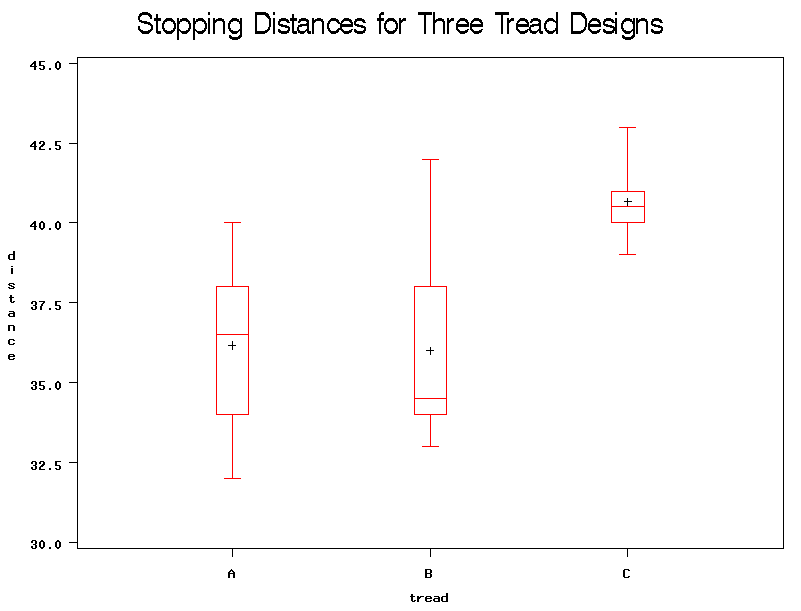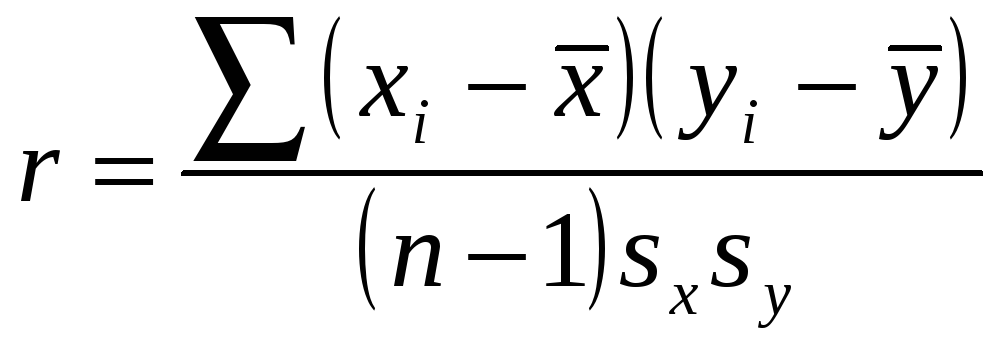# Ch 3 – Descriptive Analysis and Presentation of Bivariate Data Definition

 Page 1/5 Date 19.05.2018 Size 249.06 Kb. #49015
1   2   3   4   5
Ch 3 – Descriptive Analysis and Presentation of Bivariate Data

Definition: When the values of two variables are measured for each member of a population or sample, the resulting data is called bivariate.
Either variable may be either qualitative or quantitative. Hence, types of bivariate data are:
1. Both variables are qualitative (attribute). Example: Gender and major are recorded for a sample of 30 college students.
2. One variable is qualitative and the other is quantitative. Example: Measured braking times for cars, when they are equipped with tires having one of three different tread designs.
3. Both variables are quantitative. Example: It is desired to relate scores on a midterm exam for 56 students in an elementary statistics class to their scores on the final exam.
Two Qualitative Variables

When both variables are qualitative, the relationship between the two may be examined by cross-tabulation, using a contingency table.

Example: A new postoperative procedure is administered to a number of patients in a large hospital. One can ask the question, “Do the doctors feel differently about this procedure from the nurses, or do they feel basically the same way?” Note that the question is not whether they prefer the procedure but whether there is a difference of opinion between the two groups. To answer this question, a researcher selects a sample of nurses and doctors and tabulates the data in table form, as shown.
 Group Prefer new procedure Prefer old procedure No preference Nurses 100 80 20 Doctors 50 120 30

One qualitative variable here is “Profession,” having the two values “Nurse” and “Doctor.” The other qualitative variable is “Opinion about new procedure,” having the three possible values “Prefer new procedure,” “Prefer old procedure,” and “No preference.” We may examine the relationship between these two variables by calculating percentages (either row percentages or column percentages). To calculate row percentages, we create a new column, called Row Total, containing the total numbers of nurses and doctors surveyed. We then divide each number in each row by the row total to get a proportion:

 Group Prefer new procedure Prefer old procedure No preference Row Total Nurses 100 (50%) 80 (40%) 20 (10%) 200 (100%) Doctors 50 (25%) 120 (60%) 30 (15%) 200 (100%)

We calculate column proportions similarly. We can also calculate cell proportions by dividing each frequency by the grand total, the combined number of nurses and doctors. It is clear from these proportions that the nurses tend to have a more favorable opinion of the new procedure than the doctors.
One Qualitative and One Quantitative Variable

When one variable is qualitative and the other is quantitative, we consider the data set to be divided into several separate sample data sets, depending on the value of the qualitative variable. Then we may do 1) graphs for each of the subsamples, and 2) numerical summary statistics for each of the subsamples.

Example: The distance required to stop a 3000-pound car on wet pavement was measured to compare the stopping capability of three different tread designs. Tires of each design were tested repeatedly on the same car on a controlled wet pavement. The resulting data are shown below.

 Stopping Distances for Three Tread Designs Design A Design B Design C 37 36 34 40 38 32 33 35 34 42 38 34 40 39 41 41 40 43

Descriptive statistics for each tread design are given below:

 Mean and Standard Deviation for Each Design Design A Design B Design C Mean Std. Dev. 36.2 2.9 36.0 3.4 40.7 1.4

The average stopping distance for Design B is lower than for the other two designs. However, there is more variability in stopping distances for Design B than for the other two designs, as we can see from the side-by-side boxplots shown below: The boxplots confirm our conclusions from the above table.Are there outlying observations in any of the three data sets? To find out, we would find the 5-number summaries for each data set, and determine whether the extreme values were within the intervals discussed in Chapter 2.

Two Quantitative Variables

When both variables are quantitative, we may represent the data set as a set of ordered pairs of numbers, (x, y). The variable x is called the input (or independent) variable; the variable y is called the response (or dependent) variable. We may examine the relationship between the two variables graphically using a scatter diagram, or scatterplot.

Example: The following data set for a sample of 6 randomly middle-age to elderly patients consists of x = age of patient, and y = measured value of systolic blood pressure of patient. We expect that as people age, their blood pressure will increase. We will examine the relationship between the two variables.

 Age, x Systolic Blood Pressure, y 43 128 48 120 56 135 61 143 67 141 70 152

To construct a scatterplot of the data using the TI-83:

1) Choose STAT, EDIT. Name one column Age; name the other column SBP.

2) Enter the data into the two columns.

3) Choose WINDOW. Set Xmin to be slightly smaller than the smallest value of x. In this case, we set Xmin = 40. Set Xmax to be slightly larger than the largest value of x. In this case, we set Xmax = 72. Set Ymin to be slightly smaller than the smallest value of y; in this case, Ymin = 118. Set Ymax to be slightly larger than the largest value of y; in this case, Ymax = 155. Set Xscl = 1, and Yscl = 1.

4) Choose 2nd, STAT PLOT. Turn Plot 1 On. For Type, choose the first type, scatterplot. For Xlist, enter the name of the x variable; for Ylist, enter the name of the y variable.

5) Hit the GRAPH key.

In this example, we see an increasing, linear trend relationship between age and systolic blood pressure, as expected. If we want to see the coordinates of the data points, we use the TRACE key.

Linear Correlation

The purpose of linear correlation analysis is to measure the strength of the linear relationship between x and y. Note: If the relationship between the two does not appear to be linear, then linear correlation analysis should not be done.

If there is an increasing linear trend relationship, so that larger values of x tend to be associated with larger values of y, then we say that there is a positive correlation between x and y.

If there is a decreasing linear trend relationship, so that larger values of x tend to be associated with smaller values of y, then we say that there is a negative correlation between x and y.

If there is no linear trend present, then we say that the correlation between x and y is zero.
Definition: Pearson’s correlation coefficient, r, is a numerical measure of the strength (and direction) of a linear relationship between two quantitative variables. The formula for the correlation coefficient is,